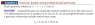# Why do we take the derivative of g(x) when using the method of

• Turion

#### Turion

Let g(x) be the right side of a heterogeneous ODE. Why do we take the derivative of g(x) when using the method of undetermined coefficients? This is to guess the form of the particular solution.

The textbook says to assume the particular solutions includes both the terms of g(x) and their derivatives but doesn't answer why.

#### Attachments

•Untitled.png
12.8 KB · Views: 413

The particular solution ##y_p## has to satisfy the differential equation. With the right value of A, when the particular solution given is subbed into the DE, the cos term will vanish to leave only sin. Thus the full 'general' form of the particular solution is this linear combination of sin and cos.

The form of this particular solution depends on the roots of the auxiliary equation from the homogeneous DE.

The particular solution ##y_p## has to satisfy the differential equation. With the right value of A, when the particular solution given is subbed into the DE, the cos term will vanish to leave only sin. Thus the full 'general' form of the particular solution is this linear combination of sin and cos.

The form of this particular solution depends on the roots of the auxiliary equation from the homogeneous DE.

For the sake of understanding, given the following problem:

$$y''-2y'+y=2f(x)\\ f'(x)=g(x)\\ f''(x)=h(x)\\ f'''(x)=i(x)\\ { f }^{ (4) }=j(x)\\ { f }^{ (5) }=k(x)\\ { f }^{ (n) }=k(x)\quad where\quad k>5$$

would the linear combination consist of f(x), g(x), and h(x) or f(x), g(x), h(x), i(x), j(x), and k(x)?

If I understand your question correctly, then I'd say f(x) and g(x) are only needed. If the RHS is already a linear combination of sin and cos then since your eqn is a second order linear DE you can solve for the particular solution, ##y_p = y_{p1} + y_{p2}## where ##y_{p1}## solves LHS = 2(Asin(kx) and ##y_{p2}## solves LHS=2(Bcos(kx)).

Here we consider two separate DE's. Consider one of the eqns above. ##y_{pi}## will be Cisin(kx) + Dicos(kx), ie a linear combo. of the original LHS and it's derivative. That is why I suggest f(x) and g(x), where g(x) was f'(x).

This is assuming that the the (possible) imaginary component of roots of the auxiliary eqn is not of the form ±ki, because then this changes the nature of the particular solution.

If I understand your question correctly, then I'd say f(x) and g(x) are only needed. If the RHS is already a linear combination of sin and cos then since your eqn is a second order linear DE you can solve for the particular solution, ##y_p = y_{p1} + y_{p2}## where ##y_{p1}## solves LHS = 2(Asin(kx) and ##y_{p2}## solves LHS=2(Bcos(kx)).

Here we consider two separate DE's. Consider one of the eqns above. ##y_{pi}## will be Cisin(kx) + Dicos(kx), ie a linear combo. of the original LHS and it's derivative. That is why I suggest f(x) and g(x), where g(x) was f'(x).

This is assuming that the the (possible) imaginary component of roots of the auxiliary eqn is not of the form ±ki, because then this changes the nature of the particular solution.

Where did you get sin and cos from?

I meant f(x) is some imaginary function. Sorry for not being clear. Basically, I am asking how many derivatives of f(x) we have to take before we stop.

Where did you get sin and cos from?

I meant f(x) is some imaginary function. Sorry for not being clear. Basically, I am asking how many derivatives of f(x) we have to take before we stop.

We do not immediately go about taking the derivative of f(x). As in the first example, if the roots of the auxiliary eqn are not ±ki, then the particular solution is a linear combination of sin and cos and so this is what you would differentiate (twice). If ±ki are roots, then the particular solution mentioned before has to be altered so as to not produce a redundant equation.

In general, for second order DE's, you have to differentiate the particular solution two times so it can then be subbed into determine the constants if initial conditions are provided. This particular solution depends on the roots of the auxiliary eqn, so it is not simply f(x).

This is the method used for undetermined coefficients. Later, you may see the method of variation of paramaters which is a more general method to find particular solutions for more complex looking f(x), but the resulting integrals may be hard to evaluate.